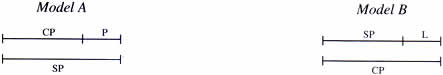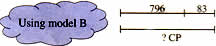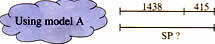Tuesday , July 5 2022# NCERT 5th Class (CBSE) Mathematics: Application Of Percentage

### Finding Selling Price

Arjun makes jewelry using semi – precious stones. He has a necklace which coasts him Rs. 1050 to make. He sells this at a profit of Rs 190 at his shop. What is the selling price of the necklace?

The earring costs him Rs. 765 to make. He sells this at a loss of Rs. 84 What is the selling price of the earrings?

When there is profit
When there is loss
Cost price of the necklace = 1050
Cost price of the earrings = Rs. 765
Profit = Rs. 190
Loss = Rs. 84
Selling price = ?
Selling price = ?
SP = CP + Profit
SP = CP – Loss
SP =  Rs. 1050 +  Rs. 190 +  Rs. 1240
SP =  Rs. 765 – Rs. 84 = Rs. 681
Check: When SP > CP there is a profit
Check: When SP > CP there is a loss
SP – CP = Profit
SP – CP = loss
Rs. 1240 – Rs. 1050 = Rs. 190
Rs. 765 – Rs. 681 = Rs. 84
Answer: The selling price of the necklace was Rs. 1240.
Answer: The selling price of the earring was Rs. 681.

## USING MODELS

All the three values related to profit and loss can be represented with help of these models.Use the models by filling in the known values. It can help us decide how to find the missing value.

(a) CP = Rs. 950
SP = Rs. 1250
P/L = ?
Rs. 1250 – Rs. 950 = Rs. 300 profitSubtract to find the difference

(b) SP = Rs. 796
L = Rs. 83
CP = Rs. ?
Rs. 796 + Rs. 83 = Rs. 879 Cost Price

Using model B(c) CP = Rs. 1438
P = Rs. 415
SP = ?
Rs. 1438 + Rs. 415 = Rs. 1853 Selling Price

Using model A